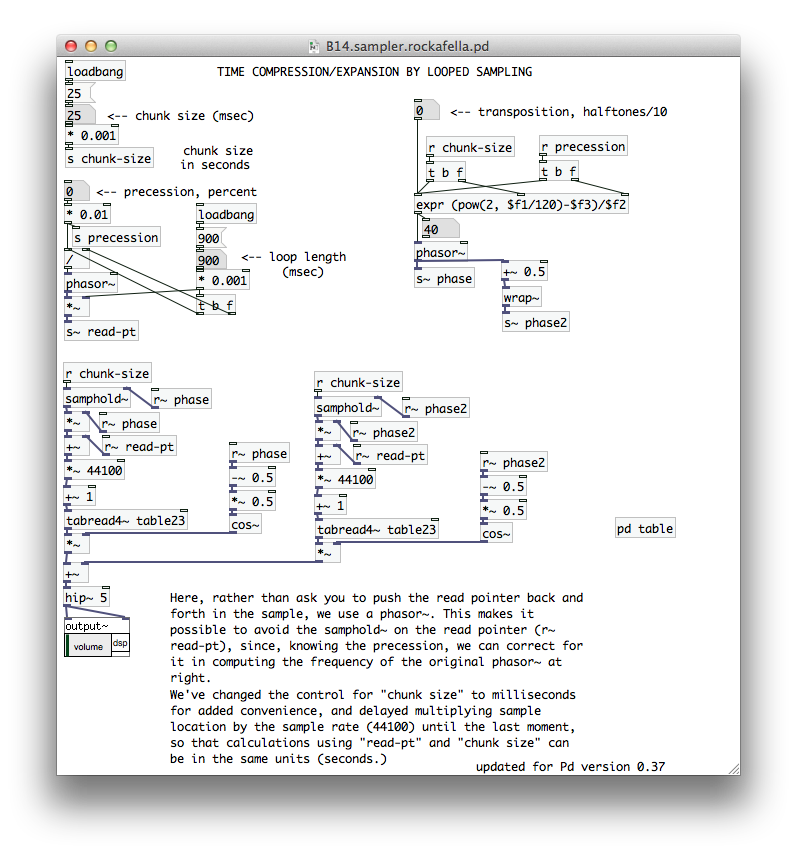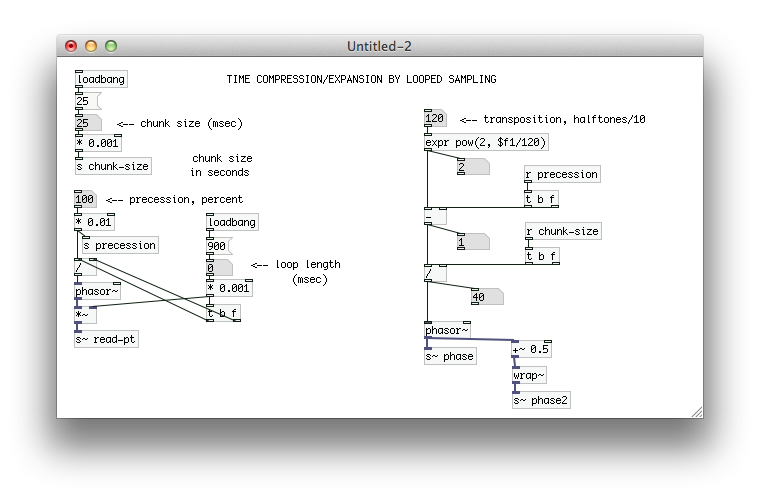• Can someone help my poor brain understand what's going on the the 'precession' and 'transposition' sections of B14.rockafella.pd?

Hi all,

I've been reading everything I can about this example and I still can't wrap my head around around why the [expr] formula looks the way it does and the reasoning behind it. What significance does 120 have in determining pitch (\$f1) and why do we subtract precession, square the quotient and divide by chunk size? Can someone smart explain to me the math behind this?

Here's a screen shot for reference:• | Posts 6 | Views 3911
• Hi rjp9,

I will have a look on this later, but as for the 120 inside the [expr], that's because the unit used here is semitone/10. Look, There are 12 semitones in an octave, meaning that to double the frequency of something you need 12 semitones. So you will find that the formula that gives the ratio between two frequencies using their difference in semitones is [expr pow(2, \$f1/12]; for instance, input a difference of 0 semitones between them and the ration becomes 1 (same frequencies), or input a difference of 12 semitones and the ratio becomes 2 (an octave, such as between 220Hz and 440Hz). So in the case above, since the unit is semitone/10 (selected to give more precision to the user, so you can also use micro intervals), there are 120 of them in each octave and the same as logic described above applies.

As for the rest, I will have a look later and see if I figure it out.

Take care,
Gilberto

• @gsagostinho Oh so in [expr pow(2, \$f1/120], the "pow 2" doesn't mean squared but rather base-2 raise to the (\$f1/120) power. For whatever reason the help files don't show that on my setup and I just assumed it meant "squared". This makes sense because for every octave you increase or decrease, your double or halve your frequency (respectively).

• Ok so I'm still stumped as to the other two operations going. I broke them up to make them easier to see:What is precession exactly and why are we subtracting it? When I listen to the example, it sounds like it sets total overall speed, but I'm not sure if that's correct. Why do we divide whatever number that is by the chunk-size?

I guess what this all boils down to and what I cannot for the life of me figure out is why are there two phasors~ and what's the relationship between the two.

• @rjp9 Miller Puckette's book is a good resource for understanding these examples http://msp.ucsd.edu/techniques/latest/book-html/node38.html

• @seb-harmonik.ar This is exactly what I needed thank you so much!

| Posts 6 | Views 3911
Internal error.

Oops! Looks like something went wrong!